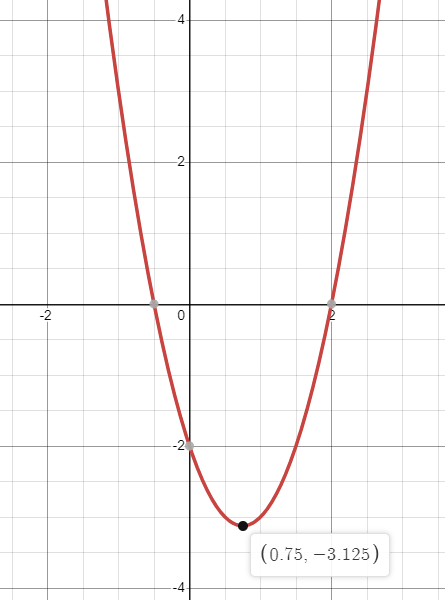When I was in school, I was taught the Quadratic Formula. I was taught that it was the most efficient, more reliable way to find the roots of a quadratic function.

This is what I was taught: Given a function in Standard Form, $$ax^2+bx+c$$, its roots can be found by evaluating $$\frac{-b\pm\sqrt{b^2-4ac}}{2a}$$.

I was instructed to commit this to memory, which I did. Decades later, as I trained to become a high school mathematics teacher, it popped back into my brain almost as readily as the so-called Pythagorean Theorem.

The thing is, I knew the Quadratic Formula, but I wasn’t entirely sure why it worked. It was something that had been foisted onto my memory cells as a frame in which to put puzzle pieces, and it would magically give me the roots.

What if there’s a better way?

A less efficient way, certainly: It has two middle steps. But a way grounded in concepts instead of in pushing letters and numbers around.

First, let’s step back for a moment and think about those two roots of a quadratic function.

Arguably the most important point on the graph of a quadratic function, even more so than the roots, is the vertex. Every quadratic function has the same basic shape, of a parabola, with a single turning point:The graph of $$y = 2x^2 -3x-2$$.

It is common to call the coordinates of this vertex $$(h, k)$$, where $$h$$ represents the $$x$$coordinate and $$k$$ represents the $$y$$-coordinate (because “h” is the first letter of “horizontal” and “k” is the first letter of “kvertical”).

We could observe that that two branches of the parabola are symmetrical: Given any random $$y$$-value, the two points of the parabola can be connected by a segment whose midpoint is on $$x = h$$.

This means that, whatever our roots are, they are the same distance from $$h$$. Let’s call this distance $$d$$, so our roots are $$h\pm d$$.

The exact formula for $$d$$ is a bit more challenging, but we can make a few more observations. Since this is a quadratic, it’s reasonable to assume $$d$$ involves a square root. Since $$a$$ is related to how steep the parabola’s legs are, it’s reasonable to assume $$d$$ involves dividing by that steepness. And since the roots are related to how far above or below the axis the vertex is, it’s reasonable to assume $$d$$ is directly related to $$k$$ somehow.

The simplest formula that satisfies those reasonable assumptions is $$d = \sqrt{\frac{k}{a}}$$.

The reality is not far off from this. There’s one small problem. In the diagram above, for instance, $$k$$ is negative and $$a$$ is positive. Dividing a negative by a positive gives us a negative, which doesn’t have a real square root.

Likewise, if $$a$$ is negative, we have two real roots only if the vertex is above the $$x$$-axis, that is, if $$k$$ is positive. So we need to account for this by taking the opposite of our quotient.

So, given our basic observations, the simplest possible formula for $$d$$ that satisfies all of our conditions is: $$d = \sqrt{-\frac{k}{a}}$$.

This formula is completely motivated by important observations about the graph of the parabola. The explanation is not a proof, but this is at least a reasonable possibility. If this pans out, that makes our quadratic formula this: $h \pm \sqrt{-\frac{k}{a}}$

This is provable. I’ll provide the proof later, but this is indeed the formula for the roots of a quadratic if we know the vertex and the stretch factor.

Con: This requires the vertex, which is not immediately evident from the Standard Form, which is what we teach as the default form of a quadratic.

Finding the vertex from the standard form involves two steps, one of which will look very familiar given the Quadratic Formula.

The Vertex Form of a quadratic function is $$a(x-h)^2+k$$. This allows us to immediately see the vertex, but it’s not the Standard Form. However: $a(x-h)^2+k=ax^2-2ahx+ah^2+k$ meaning that $$b=-2ah$$ and hence $$h=-\frac{b}{2a}$$.

From here, we have two choices. We could solve $$ah^2+k=c$$ for $$k$$, or we could plug $$h$$ into the function and evaluate for $$k$$, that is, $$k=ah^2+bh+c$$. Students usually prefer to do that latter, although I’ll do the former in my proof below.

So that gives us three steps for finding the roots of a quadratic in Standard Form:

1. Determine $$h$$.
2. Determine $$k$$.
3. Evaluate $$h\pm\sqrt{-\frac{k}{a}}$$.

This involves three steps instead of one, but it has several definite advantages. First, it also gives us the vertex of the parabola, something we’d need to evaluate separately otherwise.

More importantly to my mind, though: we’ve replaced an onerous, difficult to explain and even more difficult to remember formula with two simpler formulas, one of which can be completely motivated by concepts and understanding what a function, and what a quadratic function, is all about.

So let’s look at the proof that our two Quadratic Formulas above are equivalent. What we need to prove first: $\sqrt{-\frac{k}{a}}=\frac{\sqrt{b^2-4ac}}{2a}$

Let’s solve $$ah^2+k=c$$ for $$k$$. Recall that $$h=-\frac{b}{2a}$$. \begin{align} k &= c – ah^2 = c – a(-\frac{b}{2a})^2 \\ &= c-\frac{ab^2}{4a^2} = c-\frac{b^2}{4a} \\ &= \frac{4ac-b^2}{4a}\end{align}

Now we’re ready to swap that out: $\sqrt{-\frac{k}{a}} = \sqrt{-\frac{4ac-b^2}{4a^2}} = \frac{\sqrt{b^2-4ac}}{2a}$

Since $$h=-\frac{b}{2a}$$, $h\pm\sqrt{-\frac{k}{a}} = \frac{-b\pm\sqrt{b^2-4ac}}{2a}$ which is what I was intending to prove.Clio Corvid

## 1 Comment

This site uses Akismet to reduce spam. Learn how your comment data is processed.